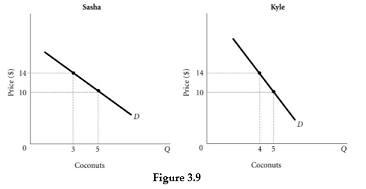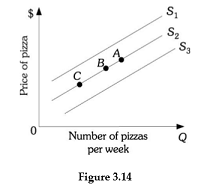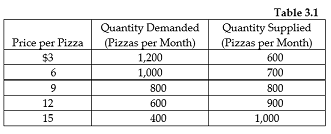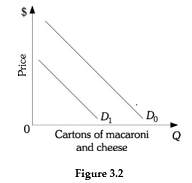# Quiz for Chapter 03【含答案】 Principles of Microeconomics

（1）单选题Refer to Figure 3.9. Assume that there are only two people in the market for coconuts: Sasha and Kyle. Along the market demand curve for coconuts, at a price of ________, quantity demanded would be ________.

A  $10; 8 B$10; 9

C  $14; 9 D$14; 7

（2）单选题Refer to Figure 3.9. Assume there are only two people in the market for coconuts: Sasha and Kyle. Along the market demand curve for coconuts, at a price of ________, quantity demanded would be ________.

A  $10; 10 B$14; 9

C  $14; 8 D$10; 9

（3）单选题Refer to Figure 3.14. A decrease in the wage rate of pizza makers will cause a movement from Point B on supply curve S2 to

A  supply curve S1.

B  Point A on supply curve S2.

C  supply curve S3.

D  Point B on supply curve S2

（4）单选题Refer to Figure 3.14. An increase in supply is represented by the movement from

A  S2 to S3.

B  Point B to Point C along supply curve S2.

C  S2 to S1.

D  Point B to Point A along supply curve S2.

（5）单选题Refer to Table 3.1. This market will be in equilibrium if the price per pizza is

A  6

B  9

C  12

D  15

（6）单选题Refer to Table 3.1. If the price per pizza is \$12, the price will

A  increase because there is an excess demand in the market.

B  decrease because there is an excess demand in the market.

C  remain constant because the market is in equilibrium.

D  decrease because there is an excess supply in the market.

（7）单选题

Which of the following will definitely occur when there is an increase in demand for and a decrease in supply of milk?

A  an increase in equilibrium quantity

B  a decrease in equilibrium quantity

C  a decrease in equilibrium price

D  an increase in equilibrium price.

（8）单选题

SCENARIO 3.2: Lettuce and spinach are substitutes. Lettuce and tomatoes are complements. Lettuce is a normal good. During the winter, about 20% of the lettuce crop was destroyed by flooding.

Refer to Scenario 3.2. As a result of the flooding during the winter, you would expect that

A  the supply of lettuce would decrease, the price of lettuce would increase, and the quantity demanded of lettuce would decrease.

B  the price of lettuce would increase and both the quantity of lettuce supplied and the quantity of lettuce demanded would increase.

C  the supply of lettuce would decrease, the price of lettuce would increase, and the demand for lettuce would decrease.

D  the price of lettuce would increase, the supply of lettuce would increase, and the quantity demanded of lettuce would decrease.

（9）单选题

SCENARIO 3.2: Lettuce and spinach are substitutes. Lettuce and tomatoes are complements. Lettuce is a normal good. During the winter, about 20% of the lettuce crop was destroyed by flooding.

Refer to Scenario 3.2. The floods that destroyed part of the lettuce crop would have caused the equilibrium price of spinach to ________ and the equilibrium quantity of spinach to ________

A  increase; increase

B  decrease; decrease

C  increase; decrease

D  decrease; increase

（10）单选题

SCENARIO 3.2: Lettuce and spinach are substitutes. Lettuce and tomatoes are complements. Lettuce is a normal good. During the winter, about 20% of the lettuce crop was destroyed by flooding.

Refer to Scenario 3.2. The government wants to protect consumers from rising food prices. Therefore, price restrictions are imposed on lettuce growers prohibiting them from raising the price of lettuce. This will cause

A  an excess demand for lettuce.

B  a decrease in the supply of lettuce.

C  an increase in the demand for lettuce.

D  an excess supply of lettuce.

（11）单选题

Which of the following is held constant along the demand curve?

A  quantity

B  price of the good

C  income

D  both A and B

（12）单选题Refer to Figure 3.2. Which of the following would be most likely to cause the demand for macaroni and cheese to shift from D1 to D0?

A  an increase in income, assuming macaroni and cheese is a normal good

B  an increase in the price of macaroni and cheese

C  an increase in the quantity demanded for macaroni and cheese

D  an increase in the price of flour used to make macaroni and cheese

（13）单选题

During an economic downturn when consumer income falls, the demand for tacos increases and the demand for sushi decreases. This implies that tacos

A  are a normal good and sushi is an inferior good.

B  and sushi are complements.

C  are an inferior good and sushi is a normal good.

D  are an economic bad and sushi is an economic good.

（14）单选题

When the decrease in the price of one good causes the demand for another good to decrease, the goods are

A  normal.

B  substitutes.

C  inferior.

D  complements.

（15）单选题

The price of mozzarella cheese, which is used in making pizza, increases. In the market for pizza you would expect that

A  the demand for pizza would decrease and the price of pizza would fall.

B  the demand for pizza would increase and the price of pizza would increase.

C  the supply of pizza would increase and the price of pizza would decrease.

D  the supply of pizza would decrease and the price of pizza would increase.